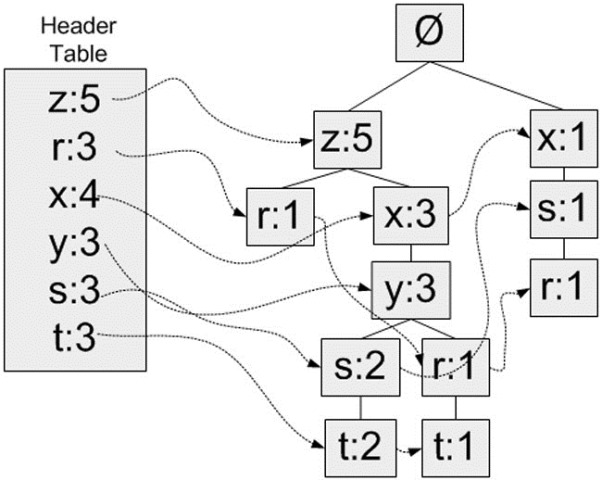# 关联分析中寻找频繁项集的FP-growth方法

 数据集ID 数据 001 r, z, h, j, p 002 z, y, x, w, v, u, t, s 003 z 004 r, x, n, o, s 005 y, r, x, z, q, t, p 006 y, z, x, e, q, s, t, m

显然这样的方式，计算量很大，当项目增多，项集的数目是指数增长的。当然我们也可以应用一些规律

1）如果一个项集是频繁项集，那么它的子集都是频繁项集

2）如果一个项集不是频繁项集，那么它的超集也不是频繁项集

Apriori算法就是应用了这些方法可以减少寻找频繁项集的计算。而FP-Growth算法则另辟蹊径，它在遍历数据的时候构造一个树结构，当树构造完成，每个节点记录的值就是这个节点到根节点路径上的项集的支持度。

 数据集ID 数据 按单元数支持度重排后的数据 001 r, z, h, j, p z, r 002 z, y, x, w, v, u, t, s z, x, y, s, t 003 z z 004 r, x, n, o, s x, s, r 005 y, r, x, z, q, t, p z, x, y, r, t 006 y, z, x, e, q, s, t, m z, x, y, s, t单元素 前缀路径 z {}: 5 r {x, s}: 1, {z, x, y}: 1, {z}: 1 x {z}: 3, {}: 1 y {z, x}: 3 s {z, x, y}: 2, {x}: 1 t {z, x, y, s}: 2, {z, x, y, r}: 1

然后对每个元素的所有前缀路径再执行一次FP树的构造过程，这样看到去除这个元素后能得到什么样的频繁项集。如下可以顺利得出{z,x,y} + {t}是一个支持度为3的频繁项集。1. https://www.cnblogs.com/qwertWZ/p/4510857.html

posted @ 2018-08-17 18:03  何振华Jeanva  阅读(1636)  评论(2编辑  收藏